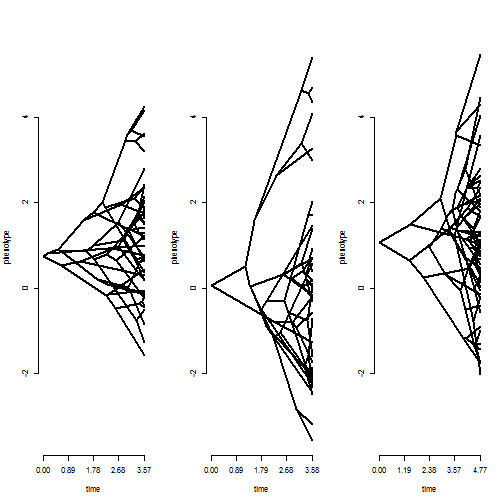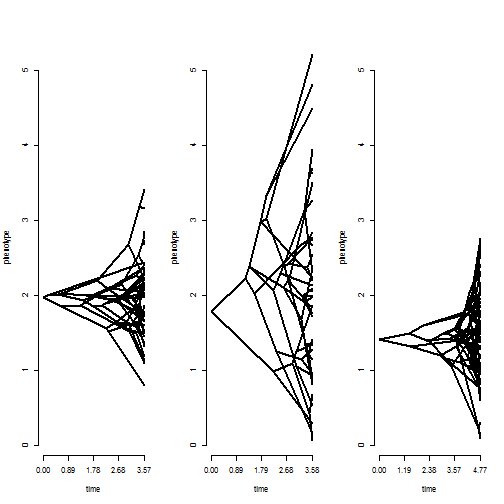## Saturday, September 23, 2017

### `ratebytree` for comparing rate (and process) of evolution between trees, now with user control of regimes

As I've mentioned in prior posts (1, 2, 3, 4, 5, 6), I'm currently in the midst of revising a manuscript describing the phytools function `ratebytree`.

In its original incarnation, this function was designed for the relatively simple task of comparing the Brownian rate of evolution between trees. More specifically, to fit two models: one in which each tree was permitted to have a different rate; and a second in which all trees had the same rate. In this form it was a very simple modification of O'Meara et al.'s (2006) censored model.

The editor & reviewers requested a number of new things, I have been diligently working to add these (summarized here). The final of these was to permit the user to specify different process regimes for different subsets of the trees in our full set. For instance, if we have 4 trees, perhaps we'd like to fit a model in which the first three have one rate, and the fourth a second.

Although this is genuinely no more complex than what I was doing already, at first I was befuddled as to how to do this without writing a completely new function to do it. Then it occurred to me that a multi-rate model in which some sets of trees have the same rate (and other sets, a different rate or different rates), is really no different from fitting a series of common-rate models and then summing the likelihood. (In practice, what I actually did was write a multi-rate function that computes the likelihood by summing multiple calls to the existing common rate function.)

I just pushed this update to GitHub.

Here I'll demonstrate it:

``````library(phytools)
packageVersion("phytools")
``````
``````##  '0.6.28'
``````
``````trees
``````
``````## 3 phylogenetic trees
``````

First, I'll fit a set of Brownian models in which trees 1, 2, & 3 all have different rates for trait x; and a model in which 1 & 3 have the same rate:

``````## our data:
par(mfrow=c(1,3))
nulo<-mapply(phenogram,tree=trees,x=x,MoreArgs=list(ylim=range(x),
ftype="off"))
````````````## fit models
fit.all<-ratebytree(trees,x)
fit.all
``````
``````## ML common-rate model:
##          s^2     a    a    a    k   logL
## value    1.2117  0.7489  0.058   1.0751  4   -223.603
## SE       0.1399  0.8292  1.1014  1.2046
##
## ML multi-rate model:
##          s^2  s^2  s^2  a    a    a    k   logL
## value    0.6709  2.1068  1.0656  0.7489  0.058   1.0751  6   -216.0332
## SE       0.1342  0.4711  0.1946  0.617   1.4523  1.1297
##
## Likelihood ratio: 15.1397
## P-value (based on X^2): 5e-04
##
## R thinks it has found the ML solution.
``````
``````fit.reg<-ratebytree(trees,x,regimes=c(1,2,1))
fit.reg
``````
``````## ML common-rate model:
##          s^2     a    a    a    k   logL
## value    1.2117  0.7489  0.058   1.0751  4   -223.603
## SE       0.1399  0.8292  1.1014  1.2046
##
## ML multi-rate model:
##          s^2  s^2  a    a    a    k   logL
## value    0.8862  2.1068  0.7489  0.058   1.0751  5   -217.4606
## SE       0.1195  0.4711  0.7091  1.4522  1.0302
##
## Likelihood ratio: 12.285
## P-value (based on X^2): 5e-04
##
## R thinks it has found the ML solution.
``````

Note that I have one fewer rate in the “multi-rate” model, but not one fewer ancestral state (`a`, `a`, and so one).

Next, I'll do the same thing - but using an OU model & character trait y as follows. Note that I can specify regime names arbitrarily. Before I just used `1` and `2`, but I can equally well use `A` and `B` as follows. Since the ancestral states correspond to trees, not regimes, they remain numbered `1`, `2`, and so on.

``````## our data:
par(mfrow=c(1,3))
nulo<-mapply(phenogram,tree=trees,x=y,MoreArgs=list(ylim=range(y),
ftype="off"))
````````````## fit models
fit.all<-ratebytree(trees,y,model="OU")
fit.all
``````
``````## ML common-regime OU model:
##          s^2     a    a    a    alpha   k   logL
## value    0.7577  1.9358  2.0289  1.4108  0.4931  5   -148.1179
## SE       0.1458  0.23    0.3051  0.2546  0.1635
##
## ML multi-regime OU model:
##          s^2  s^2  s^2  a    a    a    alp  alp  alp  k   logL
## value    0.5385  0.8383  1.1763  1.9196  1.7908  1.4349  1.1558  -0.016  1.7167  9   -126.9808
## SE       0.1952  0.1875  0.4359  0.0919  0.9161  0.0939  0.4753  0       0.6696
##
## Likelihood ratio: 42.2742
## P-value (based on X^2): 0
##
## R thinks it has found the ML solution.
``````
``````fit.reg<-ratebytree(trees,y,model="OU",regimes=c("A","B","A"))
fit.reg
``````
``````## ML common-regime OU model:
##          s^2     a    a    a    alpha   k   logL
## value    0.7577  1.9359  2.0289  1.4109  0.4931  5   -148.1179
## SE       0.1458  0.23    0.3051  0.2546  0.1635
##
## ML multi-regime OU model:
##          s^2[A]  s^2[B]  a    a    a    alp[A]  alp[B]  k   logL
## value    0.8731  0.8383  1.9154  1.7908  1.4312  1.4913  -0.0046 7   -128.6895
## SE       0.23    0.1875  0.0957  0.9161  0.0906  0.4256  0
##
## Likelihood ratio: 38.8568
## P-value (based on X^2): 0
##
## R thinks it has found the ML solution.
``````

The data were simulated as follows for this example:

``````trees<-c(pbtree(n=50),pbtree(n=40),pbtree(n=60))
x<-list(fastBM(trees[],sig2=1),
fastBM(trees[],sig2=2),
fastBM(trees[],sig2=1))
y<-list(fastBM(trees[],model="OU",alpha=2),
fastBM(trees[]),
fastBM(trees[],model="OU",alpha=2))
``````

I haven't implemented this for discrete characters yet, but this will have to be my next project!